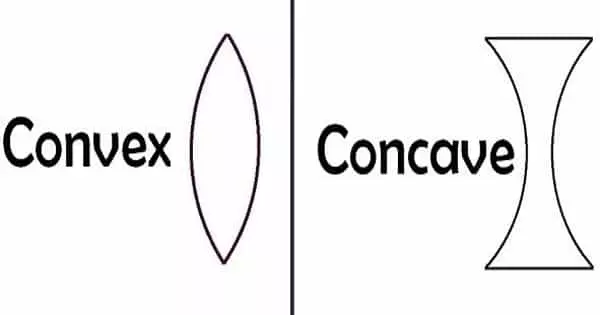Physics

# Difference between Convex and Concave LensA lens is an optical tool that converges and diverges a light beam through refraction. A lens is defined as a curved, transparent piece of glass or plastic that focuses and refracts light rays in a specific way. The curvature of the object determines how far light is bent and in which direction it is bent. They are commonly found in spectacles, microscopes, and telescopes.

The lens is classified as convex or concave based on its shape. The former brings the parallel beam of light together, while the latter disperses it. A convex lens concentrates light rays to a single point, whereas a concave or diverging lens diverts light rays. A lens is a transparent material with a curved or flat surface that is based on refraction principles. Concave and convex lenses are frequently used together to form the Concave – Convex lens. When these lenses are combined, sharper images are produced.

On passing the light through the convex lens, it bends the light rays towards each other (i.e., it converges the rays). On passing the light through the concave lens, it bends the light rays away from each other (i.e., it diverges the rays).

Difference between Convex and Concave Lens –

CONVEX LENS

• A convex lens is one that merges the light rays that pass through it at a specific point. A convex lens is one that merges light rays at a specific point as they pass through it.
• Convex lenses are those that feel larger in the center than at the edges. The lens’s curve is outward, and as light beams pass through it, it refracts and brings them together, resulting in light convergence, which is why it is also known as a converging lens.
• When the light rays pass through the convex lens, it converges the light rays and focuses on one point.
• The structure of convex lens is like, thicker at the centre and thinner at the edges. The focal length of a convex lens is positive. Due to the thicker centre of convex lenses, the objects are seen larger and closer.
• Generally, a convex lens forms a real image, but it can also create a virtual image when the object is in the middle of the focus and optical centre.

CONCAVE LENS

• A concave lens is one that disperses the light rays that hit the lenses. As a result, the lens that disperses the light rays that hit the lenses is referred to as a concave lens.
• Concave lenses are those that are narrower in the center than they are at the edges. A diverging lens is defined by the shape of a concave lense, which is round inward and bends the beams outward, causing divergence of the rays of light falling on it.
• When the light rays go through the concave lens, it diverges the beams, i.e. they spread out.
• The concave lenses are thinner at the centre and thicker at its edges, in structure. Its thin centre causes the object to look farther and smaller. The focal length of a concave lens is negative.
• The image formed by the concave lens is erect, virtual and smaller, than the object.